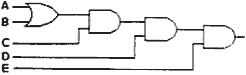Wednesday , September 28 2022# 11th CBSE NCERT Computer Science Mid Term Exam Set A

## 11th Class CBSE NCERT Computer Science: Mid Term Examination Set – A (2019-20)

Time: 3 Hrs.
Marks: 70
Class: 11th CBSE (NCERT)
Date: 20/9/2019

## General Instructions:

1. All the questions are compulsory.
2. There are 4 sections in the paper A, B, C & D.
3. Write down the question number & sub part number carefully while attempting it.

### Section A: 11th CBSE NCERT Computer Science [30 Marks]

#### (a) Predict the output of the following: 

i. x, y = 2, 6
x, y, x = y, x+2
print (x, y)

ii. a, b, c = 0.1
d=4
e=a+b-c-d
f=a+b+c*a
print(e)
print(f)

iii. a = int(input(“enter first no”))
b = int(input(“enter second no”))
a, b=b, a print(“a=”, a)
print(“b=”,b)
#if user is entering 25 and then 50

#### (b) Find out the error of the following code fragment: 

i. temp==90
print(“temp”)

ii. a,b;c=2,8,9,4
print a,b,c
c,b,a=a,b,z
print(a;b;c)

#### (f) What will be the output of the following? 

a. a=3-4+10
b. (6+4)*10+(2**5)

#### Question: 4. Answer the following questions: 

1. What are the advantages of Python Programming Language?
2. In how many different ways we can work of Python?

#### Question: 5. Write Python program for the following: 

1. Write a program to calculate the area of a circle, rectangle and triangle.
2. Write a program to ask for following as input in different lines. Enter your first name, last name, date of birth, month, day and year.

### Section B [15 Marks]

#### Question: 8. Convert the following: 

1. (4A)16 = (________)2
2. The representation of octal number (532.2)8 in decimal is ______
3. (10111001.1100)2 = () 8
4. (1234)8= ()16

#### Question: 9. Derive the Boolean expression for the logic circuit shown below:#### Question: 10.

1. Add binary numbers 111.01 and 111.01 11. 
2. Draw the logic circuit from the following expression A’B+A’C with truth table 

### Section C [15 Marks]

#### Question: 15. Answer the following questions: 

1. Differentiate between Primary Key and Unique constrain. 
2. What is the use of Alter command? 
3. Briefly Explain the following terms:  (i) Check   (ii) Update   (iii) Degree    (iv) Foreign Key
4. What is NULL value? 

#### Question: 18. Write SQL query for i to iv: 

Field
Type
Constraint
SCode
SName
No Of Member
SSymbol
Varchar(5)
Varchar(20)
Vaechar(20)
Integer
Chart(1)
Primary Key
Unique

Not Null

1. To create a table “SPORT” with the following structure.
2. Suggest suitable primary key for the above table.
3. To add a column rank with suitable data type.

## Introduction To Python: 11th Computer Science Chapter 01

Chapter Name: Introduction To Python [Chapter 01] Class: 11th Subject: Computer Science 1.1 Introduction: General-purpose …